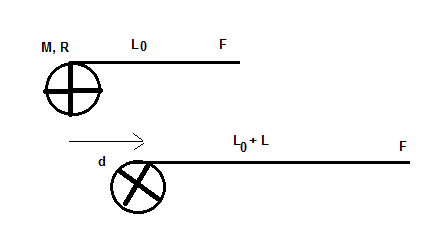# A string is wrapped around a disk of mass M and radius R. The initial length of the string is...

## Question:

A string is wrapped around a disk of mass {eq}M {/eq} and radius {eq}R {/eq}. The initial length of the string is {eq}L_0 {/eq}. Starting from rest you pull the string with a constant force {eq}F {/eq} along a nearly frictionless surface. At the instant when the center of the disk has moved a distance {eq}d {/eq}, a length {eq}L {/eq} of the string has unwound from the disk. At this instant, what is the angular speed, {eq}\omega {/eq}, of the disk about its center of mass? The moment of inertia of the disk is {eq}I = \dfrac 1 2 M R^2 {/eq}.A) {eq}\omega = \dfrac 2R \sqrt {\dfrac {Fd}{M}} {/eq}.

B) {eq}\omega = \dfrac 2R \sqrt {\dfrac FM (L+ d)} {/eq}.

C) {eq}\omega = \dfrac 2R \sqrt {\dfrac {FL}M} {/eq}.

D) {eq}\omega = \dfrac {2 \pi}R \sqrt {\dfrac {FL}M} {/eq}.

E) {eq}\omega = \dfrac 2R \sqrt {\dfrac FM \dfrac {2 \pi R}{d L}} {/eq}.

## Work-Energy Theorem:

When the body moves with velocity v from one location to another location then net work done by the force is equal to the change in kinetic energy of the body. Mathematically, we can write

{eq}\text {Change in kinetic energy = Work done} \\ \dfrac {1}{2}m(v_f^2-v_i^2)=F\times d {/eq}

Where;

• m is the mass of the body
• v is the velocity of the body
• Fis the applied force
• d is the displacement of the body in the direction of the force

Become a Study.com member to unlock this answer! Create your account

Given data

• M be the mass of the disk
• R be the radius of the disk
• F be the applied force
• d be the distance moved by the centre of mass
• L be the...Work-Energy Theorem: Definition and Application

from

Chapter 8 / Lesson 7
25K

Work and energy are closely related in physics. In this lesson, you'll learn what that relationship is as well as how we can apply it to various situations.